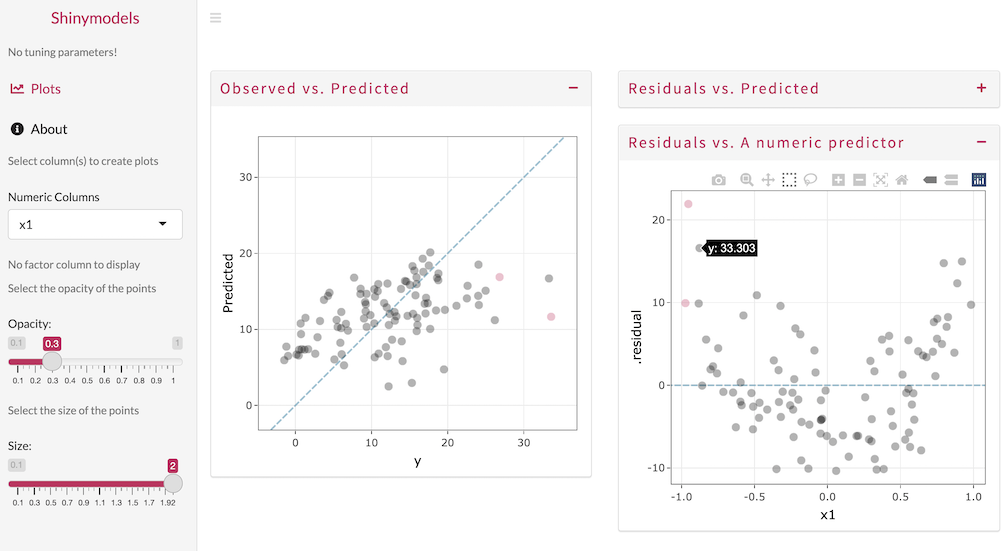The goal of shinymodels is to launch a Shiny app given tidymodels’ tuning or resampling results, to make it easier to explore the modeling results.

## Installation

You can install the released version of shinymodels from CRAN with:

install.packages("shinymodels") 

And the development version from GitHub with:

# install.packages("devtools")
devtools::install_github("tidymodels/shinymodels")

## Example

Start by tuning or fitting to resampling folds, using tune functions like fit_resamples() or tune_bayes().

As an example, we will simulate a simple relationship:

library(shinymodels)
library(tidymodels)
tidymodels_prefer()

set.seed(1)
n <- 100
simulated <-
data.frame(x1 = runif(n, min = -1), x2 = runif(n)) %>%
mutate(y = 3 - 5 * x1 + 15 * x1^2 +  + 10 * x2 + rnorm(n, sd = 5))

Let’s resample a linear regression model that is missing an important nonlinear term (i.e., poly(x1, 2)):

set.seed(2)
folds <- vfold_cv(simulated)

reg_res <-
linear_reg() %>%
fit_resamples(y ~ .,
resamples = folds,
control = control_resamples(save_pred = TRUE))

To interactively assess the model fit, we can use the explore() function:

explore(reg_res)

Use the Shiny app to explore the model results and detect any outliers or problematic observations. In the image below, the observed and predicted values are visualized, with one sample selected and highlighted. The residuals are also plotted against x1 and the quadratic pattern shows that a nonlinear term should be added.The explore() function can be used with objects produced by fit_resamples(), last_fit(), or any of the tune_*() functions.

## Contributing

This project is released with a Contributor Code of Conduct. By contributing to this project, you agree to abide by its terms.